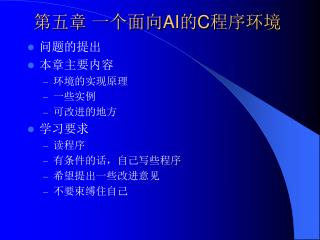Download Presentation第五章 一个面向 AI 的 C 程序环境 - PowerPoint PPT Presentation

Download Presentation第五章 一个面向 AI 的 C 程序环境
An Image/Link below is provided (as is) to download presentation

Download Policy: Content on the Website is provided to you AS IS for your information and personal use and may not be sold / licensed / shared on other websites without getting consent from its author. While downloading, if for some reason you are not able to download a presentation, the publisher may have deleted the file from their server.

- - - - - - - - - - - - - - - - - - - - - - - - - - - E N D - - - - - - - - - - - - - - - - - - - - - - - - - - -
Presentation Transcript

1. 第五章 一个面向AI的C程序环境 • 问题的提出 • 本章主要内容 • 环境的实现原理 • 一些实例 • 可改进的地方 • 学习要求 • 读程序 • 有条件的话，自己写些程序 • 希望提出一些改进意见 • 不要束缚住自己

2. 一些基本概念 • 原子 • 文字原子(符号)：abc, sin 数字原子：15, 12.3 • 表 • (a b c), (10 20 30), (a (1 23)), ((a b) (c d)) • S-表达式 原子和表统称为S-表达式 • 表头和表尾 (a) ：头=a，尾=NULL/( ) (a b c)：头=a，尾=(b c) ((a b) (c d)) ：头=(a b)，尾=((c d))

3. 表头 表尾 a a b 表结构示意图 基本结构： 例：(a), 头=a，尾=( ) 例：(a b), 头=a，尾=(b)

4. 10 a 20 b 10 a 20 b 例：((a b) (10 20)), 头=(a b)，尾=((10 20)) ((10 20)) (10 20) (a b) 例：((a b) 10 20), 头=(a b)，尾=(10 20) (10 20) (a b)

5. 基本数据结构 typedef struct node /* 节点结构 */ { char mark; /* 无用单元收集标志，暂不说明 */ union { struct node *node_ptr; char *string; int i; float f; } head; /* 表头 */ union { struct node *t; P_FUNC p; } tail; /* 表尾 */ char type; /* 类型标记 */ } NODE; type域说明 f: head.f为符点数 i: head.i为整数 c: head.string为常量字符串指针, tail.t为属性表 v: head.string为变量字符串指针, tail.t为属性表 l: 节点为表, head.node_ptr为表头, tail.t为表尾 p: tail.p为函数指针, head.string为函数名

6. …... …... …... …... 内存管理 node_buff[NODE_BUFF_NUM]: node区数组 node_num_in_buff: 一个node区含有的单元数 free_node_list: 自由单元表 string_buff: 文字原子名存储空间 abc\0def\0sin\0sum\0 … string\0 string_buff_ptr string_buff_end

7. 无用单元收集 • 为什么进行无用单元收集 • 局部变量引起 • 变量指针改变 例：p指向(a b c)改为指向(d e f)后，如果再没有其他指针指向(a b c)，则(a b c)成为无用的单元 • 如何知道哪些单元是无用的？ • 所有符号单元 • 所有符号的属性 • 所有正在使用的“NODE *”型变量所指向的内容

8. 无用单元收集方法 • NODE **var_list[VAR_LIST_LEN]：变量堆栈 • 标记： 0：自由单元 1，2：交替使用，标记一个单元被收集 • 通过free_node函数启动无用单元的收集过程。 • 建立一个单元的过程(new_node函数)： • 有自由单元，则申请一个 • 无自由单元，则启动无用单元收集，申请一个

9. 建立S-表达式的手段 • 读串： • sread_s(“(a b c)”) => (a b c) • 读键盘： • read_s( ) => 键盘的输入转化为s-表达式 • 读文件： • fread_s(pFile) => 从pFile所指的文件得到一个s表达式 • 注意：该文件必须以二进制形式打开 • 例：pFile = fopen(<file name>, “rb”); • make类函数： • make_atom(<string>): <string>可以是“abc”, “12.3”等 • make_constant(<string>): <string>必须是字母开头的串 • make_int(<int>): <int>是整数 • make_float(<float>): <float>是符点数

10. 一些常用函数 假定：x = (a b (c d)), y = (1 2 3 4), z = f cons(z, x) => (f a b (c d)) cons(x, y) => ((a b (c d)) 1 2 3 4) append(x, y) => (a b (c d) 1 2 3 4) // 注意：副作用 list_copy(x) => (a b (c d)) car(x) => a cdr(x) => (b (c d)) nth(1, x) => b nth(2, x) => (c d) nthcdr(2, y) => (3 4) list2(z, x) => (f (a b (c d))) read_s系列

11. 一些常用函数（续） print_s系列 print_s(NODE *s) sprint_s(char *str, NODE *s) fprint_s(FILE *pf, NODE *s) eql与equal eql：当两指针相等，或二者同为数值时，数值相等时，eql为 真，否则为假 equal：当二者逻辑上相等时，equal为真，否则为假 例：设x=(a b c); y=copy_list(x), z=x; u=a; v=a; eql(x, y) => FALSE eql(x, z) => TRUE eql(u, v) => TRUE equal(x, y) => TRUE equal(x, z) => TRUE

12. 函数调用 NODE *funcall (NODE *function) function : (<C函数名> <参数1> <参数2> ...) 返回值：该C函数的返回值 C函数的定义形式： NODE *<C函数名>(NODE *p) 声明：declare_func(<C函数名>);

13. 函数调用举例 NODE *sort (NODE *list) { // 功能：对list中的元素排序 } 声明：declare_func(sort); 设：f = (sort (3 2 4 5 1)) 则：funcall(f) => (1 2 3 4 5) 过程：funcall函数将((3 2 4 5 1))作为参数传递给sort函数

14. 符号的属性 • 任一符号可以有多个属性，每个属性可以有一个值 • 属性类似于C中的结构，但是属性的多少是动态的，随时可以改变 • 属性以A-表的形式存储 • A-表： ((<属性1> <值1>) (<属性2> <值2>) ...) • 取属性值与赋属性值 get_property(<symbol>, <property>); set_property(<symbol>, <property>, <value>);

15. 使用上的限制条件 • 在C的主函数main()中必须运行初始化函数init(); • “NODE *”型变量必须有初值； • 在函数定义的变量说明之后，必须调用GC_init; • 在函数定义中，所有的“NODE *”型变量，必须紧跟在GC_init之后，使用GC_push将其放入变量堆栈中； • C中的所有的return语句，必须用GC_return代替； • 例： NODE *Apply(NODE *pE, NODE *pS) { NODE *sPtr =NULL; GC_init; GC_push(pE); GC_push(pS); GC_push(sPtr); // 函数体 GC_return(sPtr);}

16. AIENV使用举例 • 合一算法 • M-C问题的求解——回溯算法实现 • M-C问题的求解——A*算法实现 • 形式微分程序 • 一个简单的确定性的专家系统